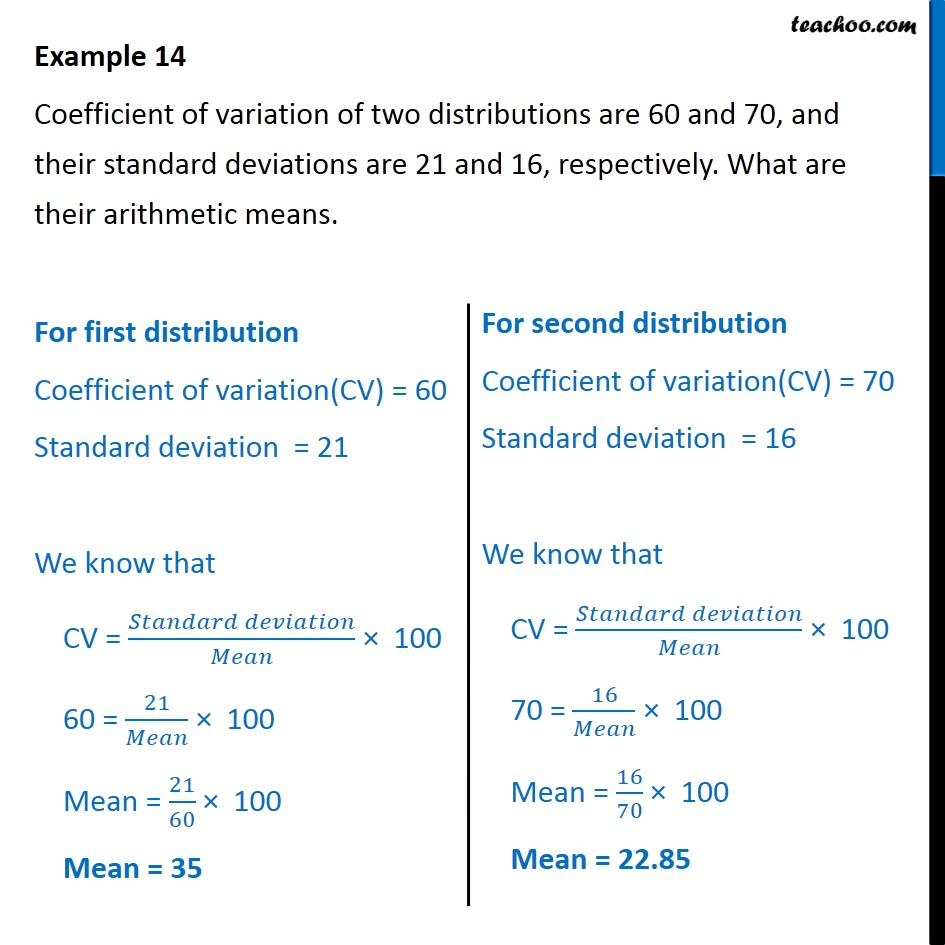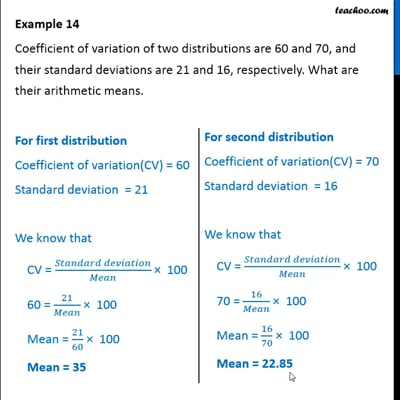Examples

Chapter 15 Class 11 Statistics (Term 1)
Serial order wiseThis video is only available for Teachoo black users

### Transcript

Example 14 - Chapter 15 Class 11 Statistics - NCERT Solution Coefficient of variation of two distributions are 60 and 70, and their standard deviations are 21 and 16, respectively. What are their arithmetic means. For first distribution Coefficient of variation(CV) = 60 Standard deviation = 21 We know that CV = (𝑆𝑡𝑎𝑛𝑑𝑎𝑟𝑑 𝑑𝑒𝑣𝑖𝑎𝑡𝑖𝑜𝑛)/𝑀𝑒𝑎𝑛 × 100 60 = 21/𝑀𝑒𝑎𝑛 × 100 Mean = 21/60 × 100 Mean = 35 For second distribution Coefficient of variation(CV) = 70 Standard deviation = 16 We know that CV = (𝑆𝑡𝑎𝑛𝑑𝑎𝑟𝑑 𝑑𝑒𝑣𝑖𝑎𝑡𝑖𝑜𝑛)/𝑀𝑒𝑎𝑛 × 100 70 = 16/𝑀𝑒𝑎𝑛 × 100 Mean = 16/70 × 100 Mean = 22.85